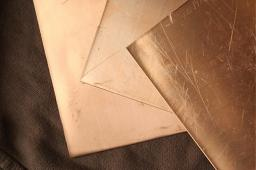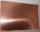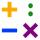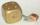# Copper plate

Calculate the thickness of the copper plate with a density 8.7 g/cm³ measuring 1.5 meters and 80 cm and its weight is 3.65 kg

h =  0.3496 mm

### Step-by-step explanation:Did you find an error or inaccuracy? Feel free to write us. Thank you!Tips to related online calculators
Do you want to convert length units?
Do you know the volume and unit volume, and want to convert volume units?
Tip: Our Density units converter will help you with the conversion of density units.
Do you want to convert mass units?

## Related math problems and questions:

• Copper sheetThe copper plate has a length of 1 m, width 94 cm and weighs 9 kg. What is the plate thickness, if 1 m3 weighs 8715 kg?
• Copper plateCopper plate length 3.2 m and width 50 cm weighs 55.42 kg. What is the plate thick, if 1 m3 of copper weighs about 8700 kg?
• Copper wireWhat is the weight in kg of copper wire 200 m long with a diameter of 0.6 cm if the density of copper is 8.8 kg?
• CuprumFrom 1600 mm long sheet of copper plate 2 mm thickness, we have separated over the whole length the belt weighing 6000 g. Calculate belt width if one dm3 copper weighs 8.9 kg.
• Cuboid 5Calculate the mass of the cuboid with dimensions of 12 cm; 0.8 dm and 100 mm made from spruce wood (density = 550 kg/m3).
• Plastic pipeCalculate the plastic pipe's weight with diameter d = 70 mm and length 380 cm if the wall thickness is 4 mm and the density of plastic is 1367 kg/m3.
• CanisterGasoline is stored in a cuboid canister having dimensions 44.5 cm, 30 cm, 16 cm. What is the total weight of a full canister when one cubic meter of gasoline weighs 710 kg and the weight of empty canister is 1.5 kg?
• Cu wireCopper wire has a length l = 820 m and diameter d = 10 mm. Calculate the weight if density of copper is ρ = 8500 kg/m3. Result round to one decimal place.
• Concrete hatchThe concrete hatch for a round well has a diameter of 1300 mm and a thickness of 80 mm. Determine its weight in kg if the density of the concrete is 2545 kg/m3
• SphereThe surface of the sphere is 12100 cm2, and the weight is 136 kg. What is its density?
• CuZnBrass is an alloy of copper and zinc. The 10 centimeter brass cube has a weight of 8.6 kg. Copper density is 8930 kg/m3, the zinc density is 7130 kg/m3. Calculate how many kg of copper and zinc a cube contains.
• The squareThe square oak board (with density ρ = 700 kg/m3) has a side length of 50 cm and a thickness of 30 mm. 4 holes with a diameter of 40 mm are drilled into the board. What is the weight of the board?
• Glass doorWhat is the weight of glass door panel 5 mm thick height 2.1 meters and a width of 65 cm and 1 cubic dm of glass weighs 2.5 kg?
• The glass1 m3 of glass weighs 2600 kg. Calculate the weight of the glass glazing panel with dimensions of 2.5 m and 3.8 m if the thickness of the glass is 0.8 cm
• Oak trunkCalculate in tonnes the approximate weight of a cylindrical oak trunk with a diameter of 66 cm and a length of 4 m, knowing that the density of the wood was 800 kg/m³.
• Iron sphereIron sphere has weight 100 kg and density ρ = 7600 kg/m3. Calculate the volume, surface, and diameter of the sphere.
• The copper wireThe copper wire bundle with a diameter of 2.8mm has a weight of 5kg. How many meters of wire is bundled if 1m3 of copper weighs 8930kg?